Courses
Courses for Kids
Free study material
Free LIVE classes
More

# Length Activities For Year 2: How to Calculate Length?## 5 Fun Activities to Calculate Length

Length activities for year 2 help to teach kids to calculate the length of any object. However, before trying different activities based on length, let us know what length is? Length is a physical dimension or measurement. It measures the distance from one point to another point. The distance can also be measured in terms of length. We can use a ruler or measuring tape to measure the length of objects.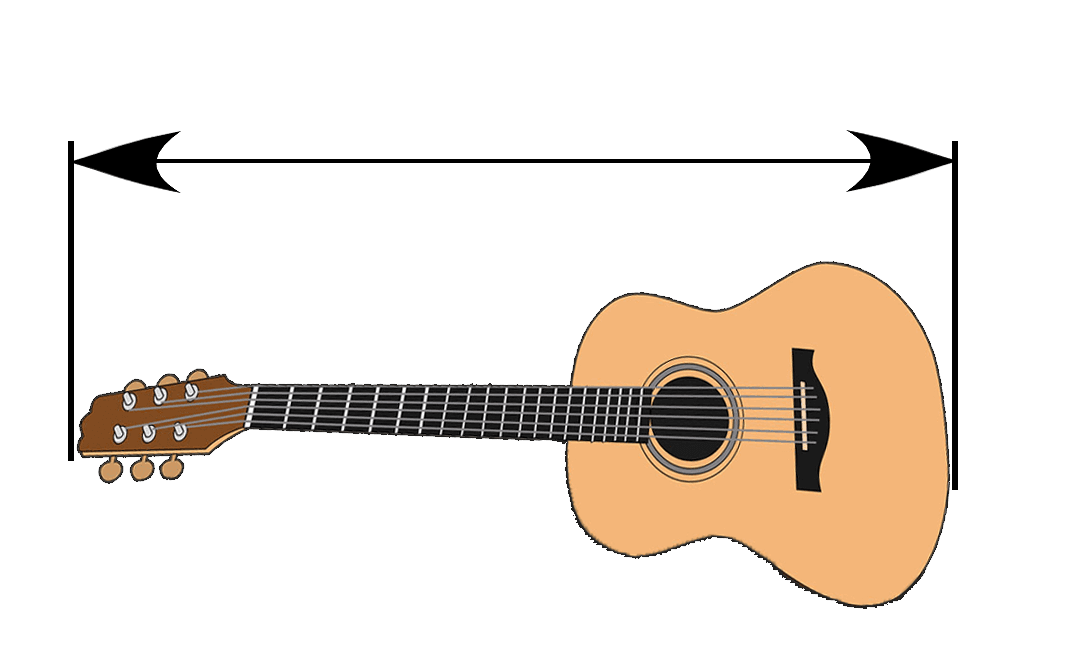The length of this guitar is one metre.

## What are the Different Units used to Measure Length?

• The unit used to measure the length of small objects is centimetre (cm). For example, pencil, postcard, notebook, etc.

• The unit millimetre (mm) is also used to measure the length of smaller objects or distances. Remember, 1 mm is equal to 0.1 cm.

• The unit used to measure objects of long length is metre (m). For example, the length of a table, the length of a cupboard, etc.

• Longer distances are measured in kilometres (km). For example, the distance between two cities.

• An inch is also a unit for measuring the length of objects. Remember, 1 inch is equal to 2.54 cm.

## Measuring Length Activities for Year 2

Let’s learn to measure the length with fun activities.

## Use Cube or Block to Measure Body Parts

Kids are always enthusiastic to know the length of their body parts like legs, hands, fingers, arms, etc. Now, you can ask your kid to measure how long their legs, hands, fingers, and arms are using different cubes or blocks. With this, they will be able to know how many long cubes their legs, hands, fingers, and arms are.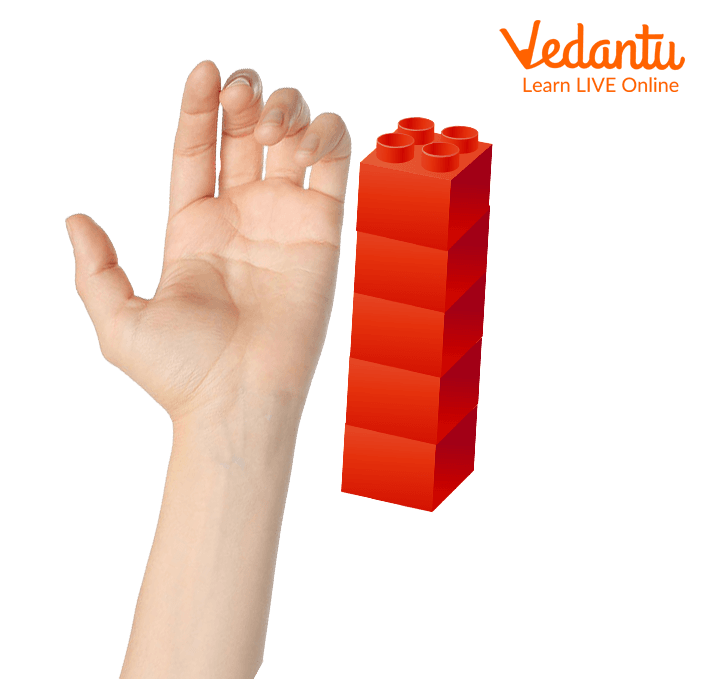Measure the length using cubes or blocks

## Comparison

Comparison is another way to ask kids to measure the length of different objects. Set a handful of objects of different lengths in front of the kids and ask them to arrange the objects according to their length.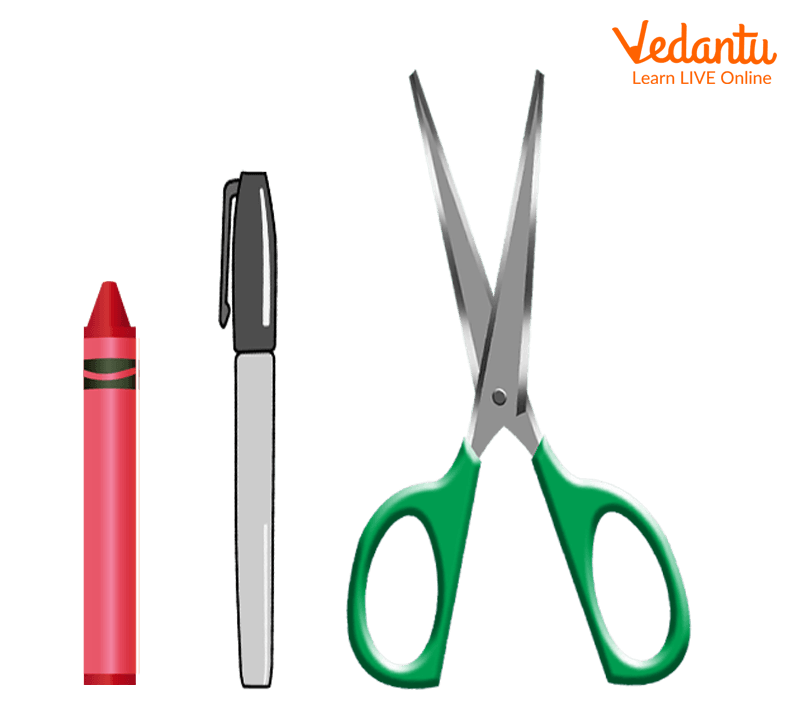Measure the length using small objects

## Using a Textbook and Ruler

This is a fun way to help your child to know what length is. Hand over different size textbooks to your kid and ask your child to measure how long the textbooks are using a ruler. Also, ask your child to record measurements of different textbooks in cm or inches.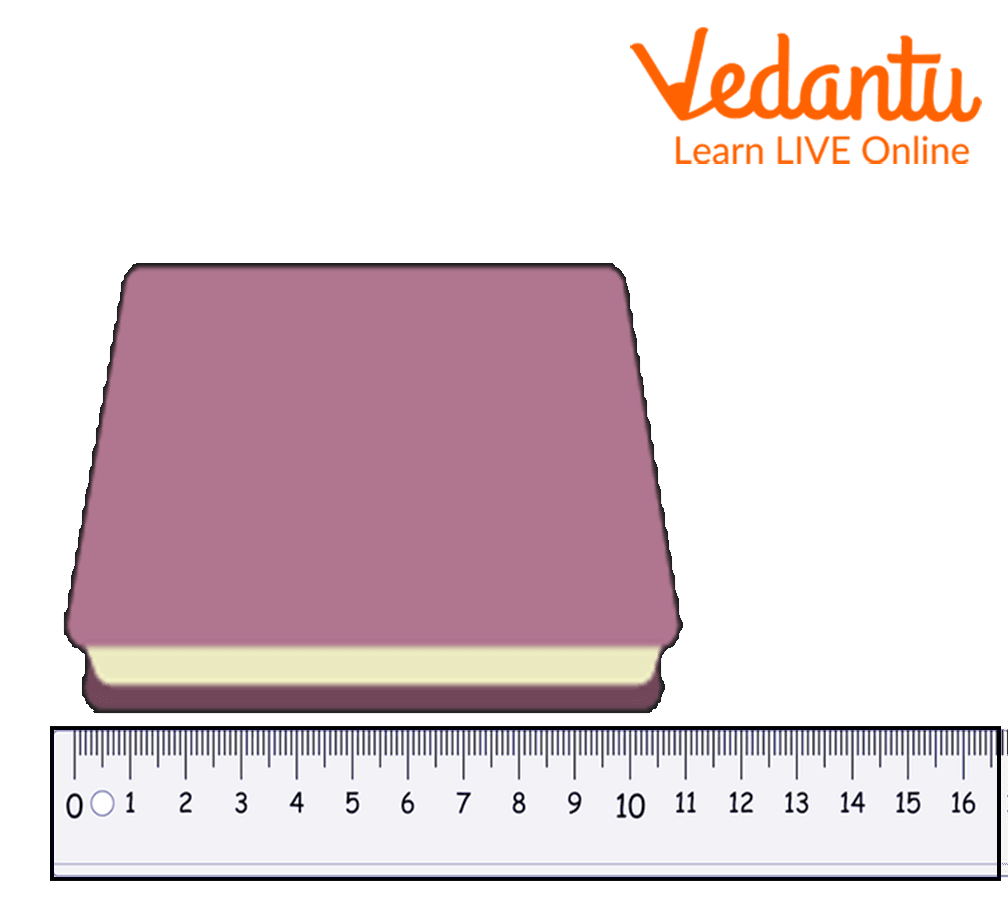Measure the length using a textbook

## Using String to Measure the Length of a Curved Line

You can ask your child to measure the length of a curved line using string. Ask your child to place a string along a curved line and tell him to mark the ending points of a curved line on the string. Now, ask your child to place a string on the ruler and measure the length of a string between marked points.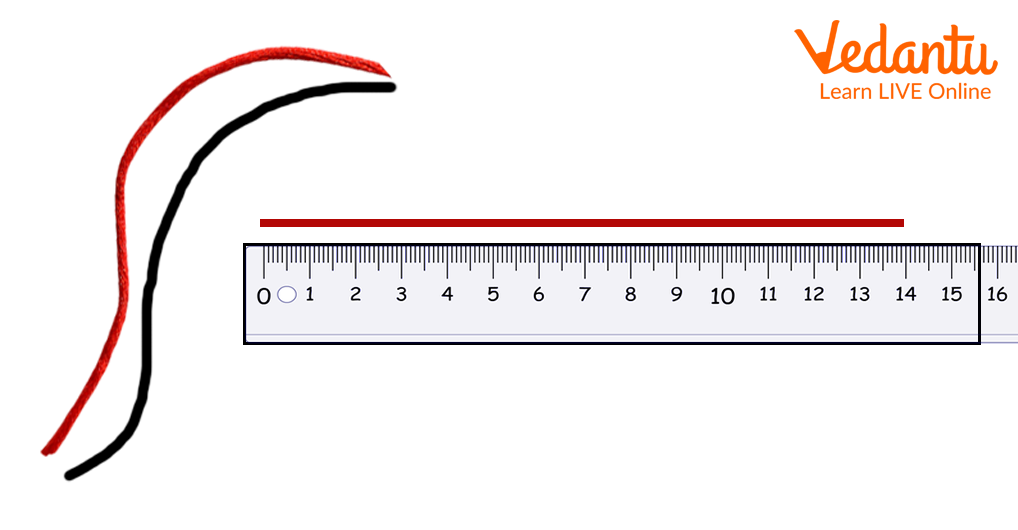Measure the length using a string

## Using Pipe Cleaners

The best idea to teach your kid what length is to cut the pipe cleaners of random lengths. Handover the pipe cleaners of different lengths to your kid and ask to measure their length. Also, ask your child to record the length of each pipe cleaner in inches and centimetres.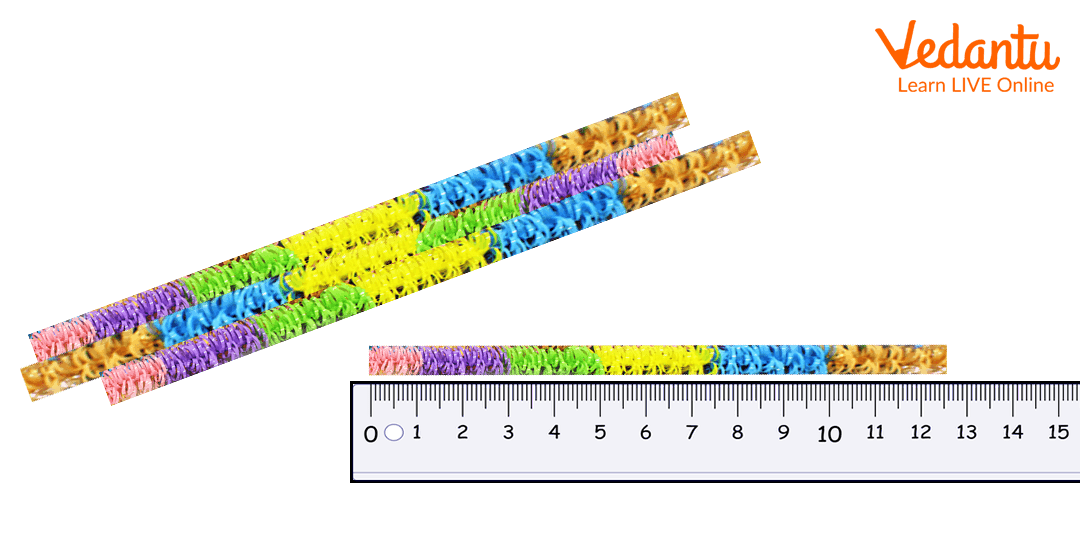Measure the length using pipe cleaners

## Practice Length Questions for Year 2

1. Calculate the length of your notebook in cm.

2. Calculate your hand length using cubes.

## Conclusion

In short, length is defined as the measurement of something from one end to another end. To enable kids to understand length, encourage them to measure the size of an object around the house or classroom using feet, hands, candies, magnetic tiles, cubes, blocks, buttons, etc.

Last updated date: 25th Sep 2023
Total views: 134.1k
Views today: 3.34k

## FAQs on Length Activities For Year 2: How to Calculate Length?

1. What is the use of length?

Length helps us to identify the size of an object or distance from one point to another. The length of an object is usually referred to as its longest side. Measuring length is very useful in real life. With length, we can know how far away a town is, the height of buildings, etc.

2. What is the basic unit of length in a metric system?

The basic unit of length in the metric system is “Metre”. All the other units of length in the metric system are derived from the metre. For example, the unit centimetre used to measure length is derived from the metre.